# Introduction

The nimbleCarbon package provides a suite of custom functions and statistical distribution for using the NIMBLE framework to fit and compare population growth models based on temporal frequencies of radiocarbon dates (Rick 1987, Williams 2012, Crema and Bevan 2021, etc.). NIMBLE is an R package that provides a system for writing Bayesian statistical models using an extensible dialect of the BUGS modelling language and a compiler that generates C++ programs for improved performance and customisation. This document provides a short guide tailored to Bayesian radiocarbon analyses via nimbleCarbon. For a general introduction to the NIMBLE framework users are strongly advised to read tutorials and manuals which can be found on NIMBLE’s official website. For an extended discussion on theoretical principles behind the proposed Bayesian approach for analysing radiocarbon frequency data see Crema and Shoda 2021.

Stable versions of the nimbleCarbon package can be installed from CRAN (with the command install.packages('nimbleCarbon')), whilst the latest development version can be obtained from GitHub using the devtools package:

library(devtools)
install_github('ercrema/nimbleCarbon')

Once the installation is completed, the package can be loaded using the library() command:

library(nimbleCarbon)
## Loading required package: nimble
## nimble version 0.12.2 is loaded.
## please visit https://R-nimble.org.
##
## Attaching package: 'nimble'
## The following object is masked from 'package:stats':
##
##     simulate
## Loading nimbleCarbon.
## Registering distrubutions and compiling functions

We will be also using the rcarbon package for processing radiocarbon dates:

library(rcarbon)

# Example 1: Exponential Growth Model

To illustrate a typical work-flow for fitting population growth models with nimbleCarbon we consider a hypothetical scenario where the target population grew with an exponential rate. More formally, we assume that the population size $$N_t$$ at time $$t$$ is given by the following equation:

$N_t = N_0(1+r)^t$

where $$N_0$$ is the initial population size at time $$t=0$$ and $$r$$ is the growth rate. The assumption of the so-called dates as data approach (Rick 1987) is that the probability of $$\pi_t$$ of observing a $$^14$$C date at time $$t$$ is proportional to $$N_t$$. It follows that that given a temporal window consisting of $$T$$ years, $$\pi_t$$ can be computed using the following equation:

$\pi_t = \frac{N_0(1+r)^t}{\sum_{t=1}^TN_0(1+r)^t}$ and because $$N_0$$ is a constant and does not affect the shape of the population curve (and hence the estimate of $$\pi_t$$), we can further simplify the equation by setting $$N_0$$ to 1. Finally, because we have to account for calibration effects we need to use index values that refer to in absolute calendar dates in BP rather than the number of years from a $$t=0$$. Once we incorporate these two factors, our equations becomes:

$\pi_{a-t} = \frac{(1+r)^t}{\sum_{t=0}^{a-b}(1+r)^t}$ where $$a$$ and $$b$$ are the calendar years in BP defining the start and the end of the time window of analysis. We can plug this equation in R and obtain a vector of probabilities for each calendar year between $$a$$ and $$b$$. For example, given $$a=6500$$, $$b=4500$$ and $$r=0.002$$ we would have:

a = 6500
b = 4500
r = 0.002
t = 0:(a-b)
pi = ((1+r)^t)/(sum((1+r)^t))
plot(a-t,pi,xlim=c(a,b),type='l',xlab='Cal BP',ylab='Probability Mass',ylim=c(0,max(pi)))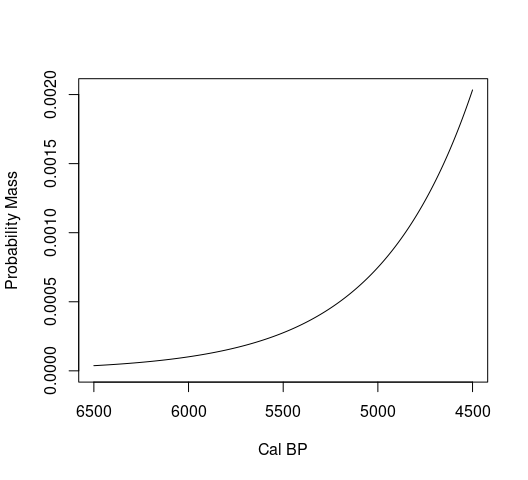Now let’s use the vector pi to generate a simulated radiocarbon data-set. This would require us to first sample calendar dates from our distribution, then back-calibrate them to $$^{14}$$C ages and assign a measurement error to each. The example below does this with 300 dates, each associated with an error of 20 years:

set.seed(123)
n = 300
calendar.dates = sample(a:b,size=n,prob=pi)
cra = round(uncalibrate(calendar.dates)$ccCRA) #back-calibrate in 14C ages cra.error = rep(20,n) #assign error of 20 years Typically, these calendar dates are calibrated and their probabilities aggregated to generate summed probability distributions (SPD). Estimates of growth rates are then obtained by fitting a regression model where the response variable is the vector of summed probabilities and the independent variable their corresponding calendar age: calibrated.dates = calibrate(cra,cra.error,verbose=FALSE) summed.prob = spd(calibrated.dates,timeRange=c(6500,4500),verbose = FALSE) summed.prob.grid = summed.prob$grid
fit <- nls(PrDens ~ exp(a + b * calBP), data = summed.prob.grid, start = list(a = 0, b = 0))
-coefficients(fit) #Estimated growth
##           b
## 0.001525565
-confint(fit,level=0.95)[2,] #95% CI 
## Waiting for profiling to be done...
##        2.5%       97.5%
## 0.001570050 0.001482002
# Note: estimates are negative because dates are in BP

However, such an approach can yield biased estimates. In the example above, the regression model suggests a growth rate of 0.00153 with a fairly narrow 95% confidence interval between 0.00148 and 0.00157 that does not include the true rate of r (see also Carleton and Groucutt 2021 for a similar analysis). Moreover, because sampling error are not accounted for, standard errors do not provide reliable measures of uncertainty, and likelihood estimates (and derived measures such as AIC) are incorrect.

A better way to conceptualise the problem and obtain unbiased estimates of $$r$$ is to consider the calendar date associated to each $$^{14}$$C sample to be a random draw from a probability distribution. In this case, the generative process becomes equivalent to the one used above to create our artificial dataset, and the core principles can be extended to any observed data and their underlying probability distributions. However, such an approach would require the calculation of the likelihood function which can be difficult if not impossible in many situations. Timpson et al (2021) have recently overcome this problem by conceptualising proposed models as probability mass functions rather than probability density functions. In other words, the insight is to treat time as discrete units so that the computation of the likelihood function becomes straightforward. In practical terms any probability distribution representing a population growth can be translated into a generalized Bernoulli distribution (i.e. a categorical distribution) where each discrete calendar year is assigned to a probability. This framing of the problem effectively allows for an inferential process similar to those employed in the Bayesian analyses of archaeological phases (Buck et al 1992) which are made available in dedicated software packages such as OxCal and BCal.

The nimbleCarbon package provides a library of probability distributions for analysing frequency distribution of radiocarbon. These are generalized Bernoulli distribution where the probabilities $$\pi_{a-1},\pi_{a-2},...,\pi_{a-b+1}$$ for each calendar year $$a-t$$ (in BP) are defined by a series of parameters that capture the demographic trajectories (i.e. the ‘shape’ of the probability distribution) within a temporal window of analyses defined by boundary parameters $$a$$ and $$b$$. Thus for example, the custom nimble distribution model dExponentialGrowth can portray our exponential growth using the parameters $$a$$, $$b$$, and $$r$$ and estimate the likelihood for any observed calendar date.

## Bayesian Inference

Now let’s analyse our simulated radiocarbon dataset by fitting the dExponentialGrowth model and estimating the population growth rate $$r$$ (we will treat our boundary parameters $$a$$ and $$b$$ as constant user-defined window of analysis). The core pipeline required for our analyses starts with the definition of our Bayesian hierarchical model via the nimbleCode() function:

model <- nimbleCode({
for (i in 1:N){
# Growth Model Likelihood
theta[i] ~ dExponentialGrowth(a=start,b=end,r=r);
# Calibration
mu[i] <- interpLin(z=theta[i], x=calBP[], y=C14BP[]);
sigmaCurve[i] <- interpLin(z=theta[i], x=calBP[], y=C14err[]);
# Measurement Error
sd[i] <- (sigma[i]^2+sigmaCurve[i]^2)^(1/2);
X[i] ~ dnorm(mean=mu[i],sd=sd[i]);
}
# Prior
r ~ dexp(1/0.0004); # Prior
})  

When carrying a Bayesian analyses of radiocarbon dates the contents required for nimbleCode() typically include four main elements. The first consist of a probability distribution (in this case dExponentialGrowth()) which defines the likelihood of observing a given calendar date (theta). The second and the third block translates such date into $$^{14}$$C Age and models its relationship to the observed data whilst accounting for measurement error of the calibration curve and the sample. For most applications these twos sections can be copied and pasted with no modifications. The final block defines the prior probability of our parameters — in this case we assign to $$r$$ a weakly informative exponential prior with a rate of 1/0.0004 (thus a mean of 0.0004), based on the average growth rate observed in several prehistoric populations (see Zahid et al 2016).

Next we define our constants and the data to be fitted. The constants will include the sample size, the calibration curve, and any other fixed parameters (in this case start and end, i.e. the window of analysis):

data("intcal20") #load the IntCal20 calibration curve, Remier et al 2020
constants <- list(N=n,calBP=intcal20$CalBP,C14BP=intcal20$C14Age,C14err=intcal20$C14Age.sigma,start=a,end=b) We then define our input data (i.e. the $$^{14}$$C ages and their associated measurement errors): data <- list(X=cra,sigma=cra.error) And finally provide a list of sensible initial parameter values to be used in the MCMC. These can be fixed: m.dates = medCal(calibrate(cra,cra.error,verbose = FALSE)) #compute the median calibrated for an initial estimate of theta if(any(m.dates>a|m.dates<b)){m.dates[m.dates>a]=a;m.dates[m.dates<b]=b} #ensure that theta is within the time range of analysis inits <- list(r=0.0004,theta=m.dates) or can be defined as series of functions generating random values (in this case from the prior) or a mixture of functions and fixed values: inits.function = function() list(r=rexp(1,1/0.0004),theta=m.dates) We are now ready to compile and run our model. The nimble package offer different options and degrees of customisation (see Nimble’s manual for details), but the quickest approach is using the nimbleMCMC() function, which requires various MCMC settings. The example below uses 10,000 iterations over 2 chains with a burn-in of 2000 steps1: mcmc.samples<- nimbleMCMC(code = model,constants = constants,data = data,niter = 10000, nchains = 2, thin=1, nburnin = 2000, progressBar = FALSE, monitors=c('r','theta'), inits=inits.function, samplesAsCodaMCMC=TRUE,setSeed=c(123,456)) ### Running Chains in Parallel The nimbleMCMC() does not run MCMC in parallel chains across different cores. However, it is possible to write a pipeline to achieve this by making use of the parallel package. The script below has been adapted from the tutorial provided on the nimble website and generates the same output as the MCMC above. # Setup parallel processing: library(parallel) ncores <- 2 cl <- makeCluster(ncores) # Generate a single wrapper function: runFun <- function(seed, data, constants, m.dates) { library(nimbleCarbon) inits.function = function() list(r=rexp(1,1/0.0004),theta=m.dates) model <- nimbleCode({ for (i in 1:N){ theta[i] ~ dExponentialGrowth(a=start,b=end,r=r); mu[i] <- interpLin(z=theta[i], x=calBP[], y=C14BP[]); sigmaCurve[i] <- interpLin(z=theta[i], x=calBP[], y=C14err[]); sd[i] <- (sigma[i]^2+sigmaCurve[i]^2)^(1/2); X[i] ~ dnorm(mean=mu[i],sd=sd[i]); } r ~ dexp(1/0.0004); }) results<- nimbleMCMC(code = model,constants = constants,data = data,niter = 10000, nchains = 1, thin=1, nburnin = 2000, progressBar = FALSE, monitors=c('r','theta'), inits=inits.function, samplesAsCodaMCMC=TRUE,setSeed=seed) return(results) } # Run the model in parallel: seeds = c(123,456) chain_output = parLapply(cl = cl, X = seeds, fun = runFun, data = data, constants=constants,m.dates=m.dates) stopCluster(cl) # Convert into a mcmc.list object for diagnostic (see below) chain_output=coda::mcmc.list(chain_output) ## Diagnostics and Posterior Distributions The argument samplesAsCodaMCMC in nimbleMCMC() ensures that the output is stored as an mcmc class object of the coda package, which offers a wide range of MCMC diagnostics. First, trace plots of the posterior samples can be plotted using the standard plot() function in R as shown in the example below: par(mfrow=c(2,1)) plot(as.numeric(mcmc.samples$chain1[,'r']),type='l',xlab='MCMC Iteration',ylab='r',main='chain 1')
plot(as.numeric(mcmc.samples$chain2[,'r']),type='l',xlab='MCMC Iteration',ylab='r',main='chain 2')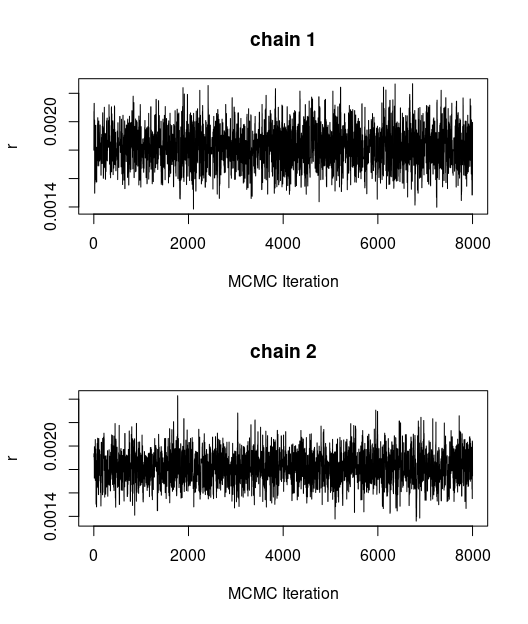If more than two chains are available, diagnostic metrics such as Gelman-Rubin convergence diagnostic ($$\hat{R}$$, Gelman and Rubin 1992) and effective sample sizes can be evaluated: library(coda) rhat = gelman.diag(mcmc.samples) head(rhat$psrf)
##          Point est. Upper C.I.
## r         1.0018125  1.0092045
## theta  1.0005038  1.0030189
## theta  1.0010134  1.0054594
## theta  1.0003518  1.0012154
## theta  1.0058999  1.0284071
## theta  0.9998822  0.9998822
ess = effectiveSize(mcmc.samples)
head(ess)
##        r theta theta theta theta theta
## 3305.452 2896.159 2982.605 3424.353 2200.403 2668.849

In this case the key parameter $$r$$ has an $$\hat{R}$$ close to 1 and a fairly large effective sample size indicating a good mixing and convergence.

We are now ready to examine our posterior. The postHPDplot() in the nimbleCarbon package offers a convenient way to display marginal posterior distributions highlighting user-defined highest posterior density interval:

postHPDplot(mcmc.samples$chain1[,'r'],rnd=5,xlab='r',ylab='Density',prob = 0.95) abline(v=r,lty=2) axis(3,at=r,label='True value of r')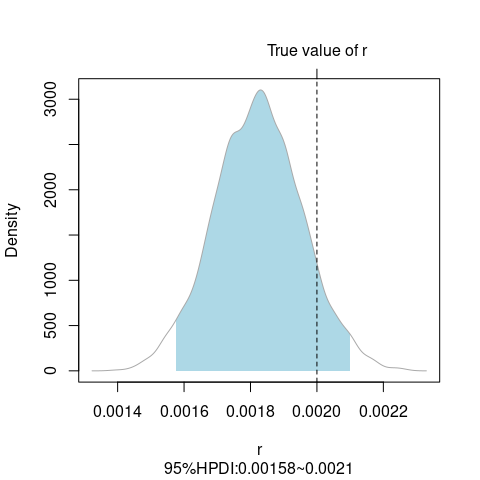In contrast to the regression based estimate used above, the 95% HPD provides a wider interval (properly accounting for sampling error) and includes the true growth rate used to generate the simulated data. While marginal posteriors can provide direct insights on the estimates of each parameter value and their associated uncertainty, more complex models with additional parameters can be harder to interpret. The modelPlot() function help visualising the shape of growth models given a list of parameter combinations. This can be used to carry out prior predictive checks (see left panel below) or to plot the fitted growth model incorporating the uncertainty of the posterior parameters (right panel below). par(mfrow=c(1,2)) set.seed(123) modelPlot(dExponentialGrowth,a=a,b=b,params=list(r=rexp(100,1/0.0004)),alpha = 0.3,ylim=c(0,0.003),main='Prior',type='spaghetti',col='lightblue') lines(a:b,pi,col=2,lty=2,lwd=2) modelPlot(dExponentialGrowth,a=a,b=b,params=list(r=mcmc.samples$chain1[,'r']),nsample=100,alpha=0.3,ylim=c(0,0.003),main='Posterior',type='spaghetti',col='lightblue')
lines(a:b,pi,col=2,lty=2,lwd=2) #true data-generating model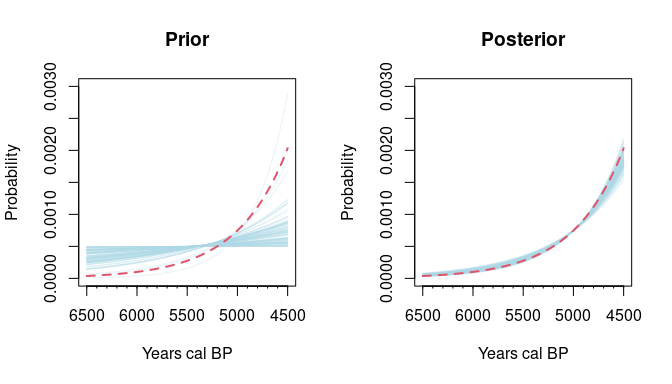# Example 2: Logistic Growth and Model Comparison

## Data Preparation

Now let’s consider a real dataset and an additional growth model. We will use a subset of radiocarbon dates from Denmark using the EUROEVOL dataset (Manning et al 2016) offered in the rcarbon package (Crema and Bevan 2021):

data(euroevol)
DK=subset(euroevol,Country=="Denmark") #subset of Danish dates
DK.caldates=calibrate(x=DK$C14Age,errors=DK$C14SD,calCurves='intcal20',verbose=FALSE) #calibration

It is common practice in the analyses of SPDs to handle potential biases arising from inter-site variation in sampling intensity by grouping dates from the same site that are close in time, summing their calibrated probabilities, and normalising the result to sum to unity (see Timpson et al 2014, Crema and Bevan 2021 for details). The resulting “bins” are then combined to generate SPDs and used as basic analytic units. This approach is not possible here, but we can achieve similar adjustment by selecting a random date from each bin using the binPrep() ad thinDates() functions in rcarbon:

# Generate bins grouping dates within 100 yrs
DK.bins = binPrep(sites=DK$SiteID,ages=DK$C14Age,h=100)
# Sample 1 date from each bin, selecting randomly the sample with the smallest error
DK.caldates = DK.caldates[thinDates(ages=DK$C14Age, errors=DK$C14SD, bins=DK.bins, size=1, thresh=1,seed=123,method='splitsample')]

We will in this case examine the density of radiocarbon dates between 7000 and 4500 cal BP. To obtain the relevant subset from DK.caldates we will use the subset() function, taking into consideration only samples with a cumulative probability equal or larger than 0.5 within the time window of analysis:

DK.caldates = subset(DK.caldates,BP<=7000&BP>=4500,p=0.5)

The SPD of the resulting subset can be visualised using dedicated function in rcarbon:

obs.spd = spd(DK.caldates,timeRange=c(7000,4500),verbose=FALSE)
plot(obs.spd)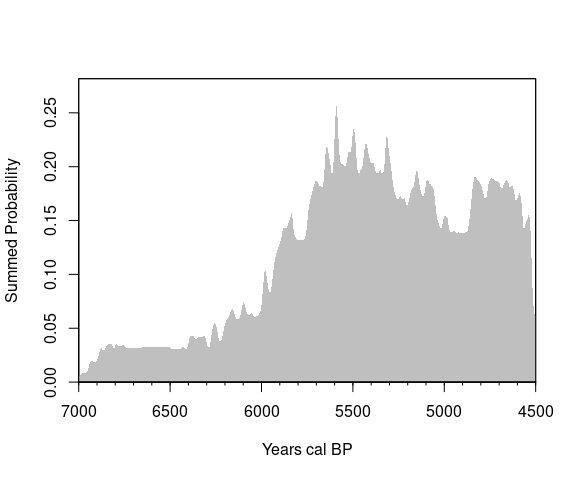Now let’s extract the $$^{14}$$C ages and their associated errors from our subset

obs.CRA = DK.caldates$metadata$CRA
obs.Errors = DK.caldates$metadata$Error

and create lists of input data and constants for our Bayesian analysis.

constants <- list(N=length(obs.CRA),calBP=intcal20$CalBP,C14BP=intcal20$C14Age,C14err=intcal20$C14Age.sigma,start=7000,end=4500) data <- list(X=obs.CRA,sigma=obs.Errors) ## Growth Models We will consider an exponential and a logistic growth model. We have already created the former: m1 <- nimbleCode({ for (i in 1:N){ theta[i] ~ dExponentialGrowth(a=start,b=end,r=r); mu[i] <- interpLin(z=theta[i], x=calBP[], y=C14BP[]); sigmaCurve[i] <- interpLin(z=theta[i], x=calBP[], y=C14err[]); sd[i] <- (sigma[i]^2+sigmaCurve[i]^2)^(1/2); X[i] ~ dnorm(mean=mu[i],sd=sd[i]); } r ~ dexp(1/0.0004); })  The logistic growth model in nimbleCarbon is defined as follows: $\pi_{a-t} = \frac{\frac{1}{1+\frac{1-k}{k}e^{-rt}}} {\sum_{t=0}^{a-b}\frac{1}{1+\frac{1-k}{k}e^{-rt}}}$ where $$k$$ is size of the population at $$t=0$$, expressed as the proportion of the carrying capacity. The numerator of the right term is a special case of the following equation: $N_{t} = \frac{K}{1+\frac{K-N_0}{N_0}e^{-rt}}$ where the carrying capacity $$K$$ is set to 1, and $$N_0=k$$. To make a nimbleCode of the logistic growth model we will use dLogisticGrowth which requires the boundary parameters a and b, the initial population size k, and the intrinsic growth rate r. Here we fix a and b to the boundary of our time-window and use exponential priors for k and r (notice that we truncate the former between 0.001 and 0.2 using the T() function in nimble): m2 <- nimbleCode({ for (i in 1:N){ # Growth Model Likelihood theta[i] ~ dLogisticGrowth(a=start,b=end,k=k,r=r); # Calibration mu[i] <- interpLin(z=theta[i], x=calBP[], y=C14BP[]); sigmaCurve[i] <- interpLin(z=theta[i], x=calBP[], y=C14err[]); sd[i] <- (sigma[i]^2+sigmaCurve[i]^2)^(1/2); X[i] ~ dnorm(mean=mu[i],sd=sd[i]); } # Prior r ~ dexp(1/0.004); # Prior k ~ T(dexp(1/0.05),0.001,0.2) })  We are now ready to define our initialisation functions for the two models so that chains have different starting parameter values for the growth models. m.dates = medCal(DK.caldates) if(any(m.dates>7000|m.dates<4500)){m.dates[m.dates>7000]=7000;m.dates[m.dates<4500]=4500} inits.function.m1 = function() list(r=rexp(1,1/0.0004),theta=m.dates) inits.function.m2 = function() list(r=rexp(1,1/0.0004),k=runif(1,0.0001,0.2),theta=m.dates) ## MCMC, Diagnostic, Model Comparison, and Posterior Predictive Check We can now use the nimbleMCMC() function again, but this time we: 1) define random seeds using the setSeed argument to ensure full reproducibility; and 2) we set the argument WAIC to TRUE so the models can be compared using the Widely Applicable Information Criterion (Watanabe 2010, Gelman et al 2014) : mcmc.samples.m1<- nimbleMCMC(code = m1,constants = constants,data = data,niter = 15000, nchains = 2, thin=1, nburnin = 3000, progressBar = FALSE, monitors=c('r','theta'), inits=inits.function.m1, samplesAsCodaMCMC=TRUE,setSeed=c(123,456),WAIC=TRUE) ## [Warning] There are individual pWAIC values that are greater than 0.4. This may indicate that the WAIC estimate is unstable (Vehtari et al., 2017), at least in cases without grouping of data nodes or multivariate data nodes. mcmc.samples.m2<- nimbleMCMC(code = m2,constants = constants,data = data,niter = 15000, nchains = 2, thin=1, nburnin = 3000, progressBar = FALSE, monitors=c('r','k','theta'), inits=inits.function.m2, samplesAsCodaMCMC=TRUE,setSeed=c(123,456),WAIC=TRUE) ## [Warning] There are individual pWAIC values that are greater than 0.4. This may indicate that the WAIC estimate is unstable (Vehtari et al., 2017), at least in cases without grouping of data nodes or multivariate data nodes. Model diagnostics and the trace plot indicates a fairly good convergence for both models par(mfrow=c(3,2)) plot(as.numeric(mcmc.samples.m1$samples$chain1[,'r']),type='l',xlab='MCMC Iteration',ylab='r',main='m1 r chain 1') plot(as.numeric(mcmc.samples.m1$samples$chain2[,'r']),type='l',xlab='MCMC Iteration',ylab='r',main='m1 r chain 2') plot(as.numeric(mcmc.samples.m2$samples$chain1[,'r']),type='l',xlab='MCMC Iteration',ylab='r',main='m2 r chain 1') plot(as.numeric(mcmc.samples.m2$samples$chain2[,'r']),type='l',xlab='MCMC Iteration',ylab='r',main='m2 r chain 2') plot(as.numeric(mcmc.samples.m2$samples$chain1[,'k']),type='l',xlab='MCMC Iteration',ylab='r',main='m2 k chain 1') plot(as.numeric(mcmc.samples.m2$samples$chain2[,'k']),type='l',xlab='MCMC Iteration',ylab='r',main='m2 k chain 2')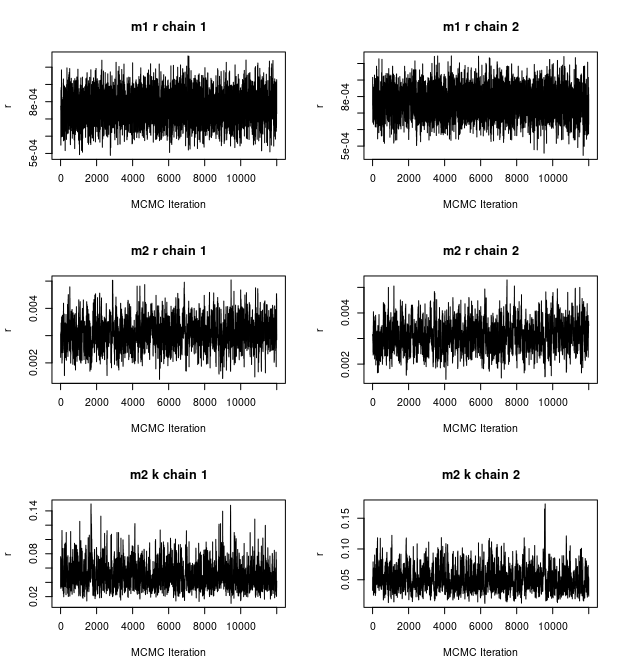m1.rhat=gelman.diag(mcmc.samples.m1$samples)
m2.rhat=gelman.diag(mcmc.samples.m2$samples) m1.ess=effectiveSize(mcmc.samples.m1$samples)
m2.ess=effectiveSize(mcmc.samples.m2$samples) head(m1.rhat$psrf)
##          Point est. Upper C.I.
## r          1.000718   1.001275
## theta   1.003431   1.003874
## theta   1.001564   1.004278
## theta   1.001474   1.004841
## theta   1.001786   1.008719
## theta   1.000460   1.002640
head(m2.rhat$psrf) ## Point est. Upper C.I. ## k 1.0062404 1.010521 ## r 1.0037537 1.003892 ## theta 1.0005100 1.002186 ## theta 0.9999535 1.000102 ## theta 1.0006073 1.000612 ## theta 1.0000517 1.000148 m1.ess ## r ## 4939.329 m2.ess[1:2] ## k r ## 1171.513 1184.580 indicating that we can have reliable marginal posterior distributions: par(mfrow=c(1,3)) postHPDplot(mcmc.samples.m1$samples$chain1[,'r'],rnd=5,xlab='r',ylab='Density',prob = 0.95,main='Model 1: r',xlim=c(0.00055,0.0055)) postHPDplot(mcmc.samples.m2$samples$chain1[,'r'],rnd=5,xlab='r',ylab='Density',prob = 0.95,main='Model 2: r',xlim=c(0.00055,0.0055)) postHPDplot(mcmc.samples.m2$samples$chain1[,'k'],rnd=5,xlab='k',ylab='Density',prob = 0.95,main='Model 2: k')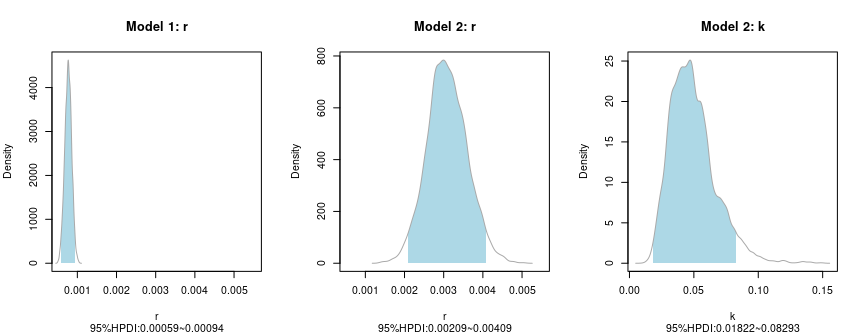We can visually compare what these parameter combinations mean in terms of population dynamics using the modelPlot() function: params.m1 = list(r=c(mcmc.samples.m1$samples$chain1[,'r'],mcmc.samples.m1$samples$chain2[,'r'])) params.m2 = list(r=c(mcmc.samples.m2$samples$chain1[,'r'],mcmc.samples.m2$samples$chain2[,'r']),k=c(mcmc.samples.m2$samples$chain1[,'k'],mcmc.samples.m2$samples$chain2[,'k'])) par(mfrow=c(1,2)) set.seed(123) modelPlot(dExponentialGrowth,a=7000,b=4500,params=params.m1,nsample=100,alpha = 0.1,ylim=c(0,0.001),main='m1: Exponential',type='envelope') modelPlot(dLogisticGrowth,a=7000,b=4500,params=params.m2,nsample=100,alpha = 0.1,ylim=c(0,0.001),main='m2: Logistic',type='envelope')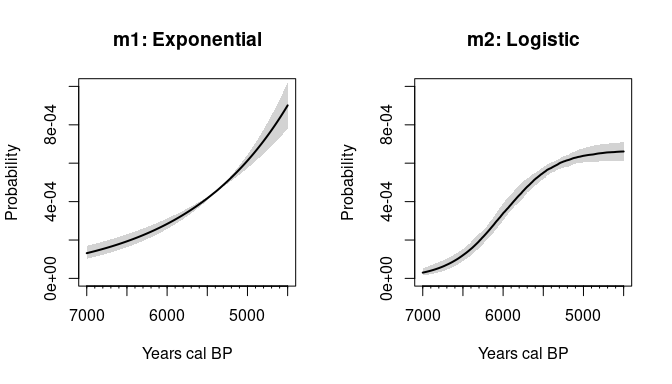The two models have different shapes, so it is worth asking which provides a better fit to the observed data. We can formally evaluate this question by comparing the WAIC values obtained from the nimbleMCMC() function. The nimbleCarbon package provide a handy function for extracting these and computing $$\Delta WAIC$$ and WAIC weights: compare.models(mcmc.samples.m1,mcmc.samples.m2) ## WAIC deltaWAIC Weights ## mcmc.samples.m1 3673.598 0.000000 0.6284222 ## mcmc.samples.m2 3674.649 1.050908 0.3715778 The results indicate a stronger support for model 2 than model 1. While this provides a relative measure of the model’s predictive performance it cannot tell whether the best model can comprehensively explain the observed temporal frequencies of radiocarbon dates. One way to evaluate the model performance in absolute (rather than relative) terms is to generate SPDs from the fitted posterior models and visually compare this to the observed SPD. The approach is similar to Monte-Carlo Null-Hypothesis Testing approach introduced by Shennan et al (2013) and implemented in rcarbon’s modelTest() function, and it is effectively a form of posterior predictive check. The postPredSPD() function in nimbleCarbon can be used to generate observed and an ensemble of fitted SPDs using the posterior samples obtained from nimbleMCMC(): set.seed(123) pp.check.m2=postPredSPD(obs.CRA,errors = obs.Errors,calCurve = 'intcal20',model = dLogisticGrowth,a = 7000,b=4500,params=list(r=mcmc.samples.m2$samples$chain1[,'r'],k=mcmc.samples.m2$samples$chain1[,'k']),method='uncalsample',nsim = 100,ncores = 3,verbose=FALSE) plot(pp.check.m2)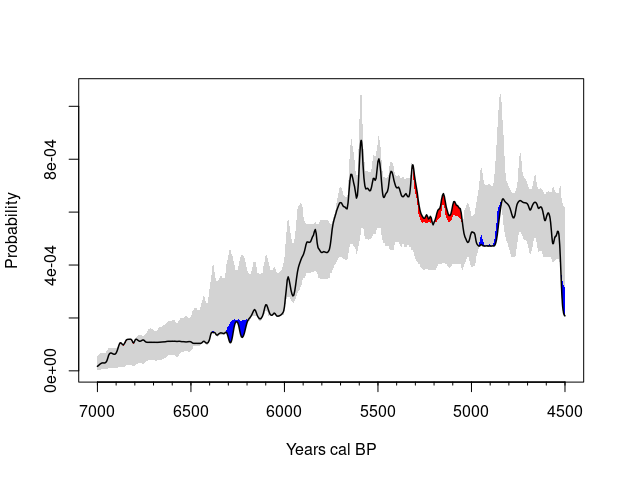In this case, although the model (grey envelope) shows generally a good fit to the data, there are some intervals where the observed density of radiocarbon dates is lower (show in blue) or higher (shown in red) than the fitted model. It is worth noting, however, that the simulation envelope does not ensure that each individual SPDs obtained from the posterior has a good fit to the data. As an additional heuristic device the correlation between the observed SPD and the SPDs generated in the posterior predictive check can be measured. The postPredCor() function can be used to extract the correlation coefficients from the output of postPredSPD() and the resulting distribution can then be examined using the postHPDplot() function: postHPDplot(postPredCor(pp.check.m2),xlab="Pearson's Correlation coefficient",ylab='Density',main='m2 goodness-of-fit',xlim=c(0,1))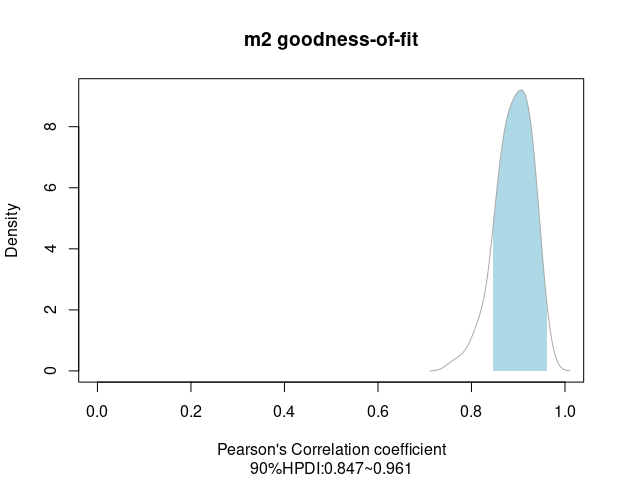In this case, the posterior SPD shows a fairly decent fit, with a relatively high range of correlation values. Notice, however, that even the SPD with the highest correlation coefficient has some discrepancies compared to the observed data due to a combination of sampling error and incorrect inference: plot(obs.spd,spdnormalised = TRUE) highest.cor.index = which.max(postPredCor(pp.check.m2)) lines(7000:4500,pp.check.m2$simmatrix[,highest.cor.index],lty=2)
legend('topleft',legend=c('observed SPD','Posterior Predictive SPD with the highest correlation'),col=c('lightgrey','black'),lwd=c(4,1),lty=c(1,2),bty='n')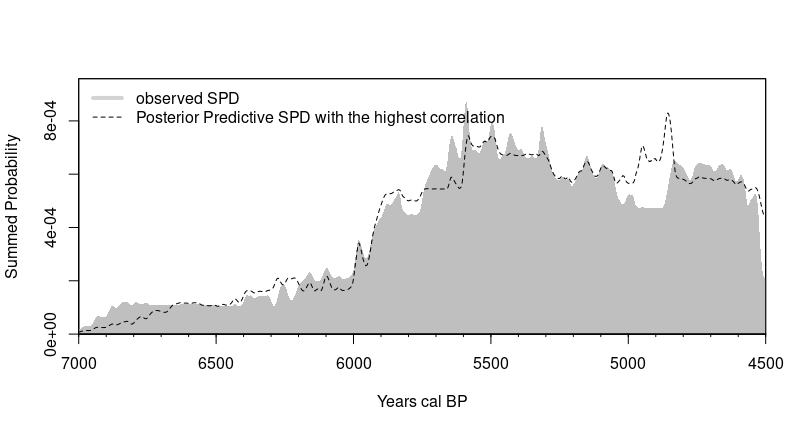# Example 3: Phase Models

The nimble framework can also be used for archaeological phase modelling. The example below illustrates this with a simulated dataset with a start date at 4500 cal BP and an end date at 3800 cal BP:

# Simulate Observed Data
a = 4500
b = 3800
set.seed(123)
n = 300
calendar.dates = sample(a:b,size=n)
cra = round(uncalibrate(calendar.dates)$ccCRA) #back-calibrate in 14C ages cra.error = rep(20,n) #assign error of 20 years # Define NIMBLE Model phasemodel <- nimbleCode({ for (i in 1:N){ # Likelihood theta[i] ~ dunif(alpha,alpha); # Calibration mu[i] <- interpLin(z=theta[i], x=calBP[], y=C14BP[]); sigmaCurve[i] <- interpLin(z=theta[i], x=calBP[], y=C14err[]); sd[i] <- (sigma[i]^2+sigmaCurve[i]^2)^(1/2); X[i] ~ dnorm(mean=mu[i],sd=sd[i]); } # Prior alpha ~ dunif(0,50000); alpha ~ T(dunif(0,50000),alpha,50000) }) #define constant, data, and inits: data("intcal20") constants <- list(N=n,calBP=intcal20$CalBP,C14BP=intcal20$C14Age,C14err=intcal20$C14Age.sigma)
data <- list(X=cra,sigma=cra.error)
m.dates = medCal(calibrate(cra,cra.error,verbose = FALSE))
inits <- list(alpha=c(3000,5000),theta=m.dates)

#Run MCMC
mcmc.samples<- nimbleMCMC(code = phasemodel,constants = constants,data = data,niter = 20000, nchains = 1, thin=1, nburnin = 5000, progressBar = FALSE, monitors=c('alpha','theta'), inits=inits, samplesAsCodaMCMC=TRUE,set.seed(123))

#Plot Posteriors
par(mfrow=c(1,2))
postHPDplot(mcmc.samples[,'alpha'],xlim=c(4600,4400),xlab='Cal BP',ylab='Posterior Probability',main='Start of Phase')
abline(v=a,lty=2)
postHPDplot(mcmc.samples[,'alpha'],xlim=c(3900,3700),xlab='Cal BP',ylab='Posterior Probability',main='End of Phase')
abline(v=b,lty=2)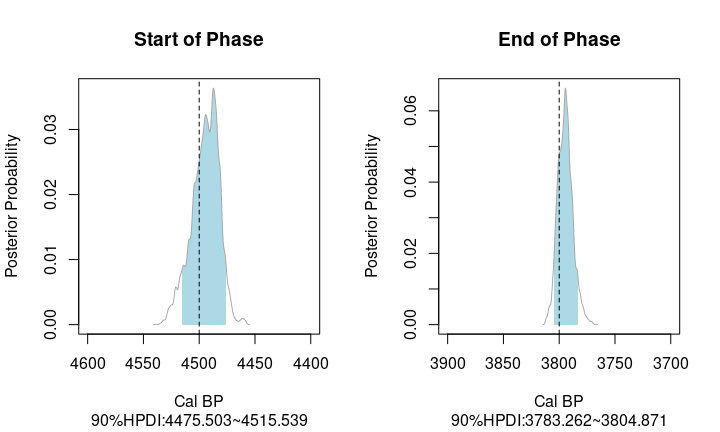While the model is able to correctly recover the true parameter values, it should be noted that for general purposes dedicated software packages such as BCal and OxCal are recommended, as they provide automatic tuning of MCMC settings as well as prior definitions and can they can easily encode more complex models with multiple phases and constraints. However, users interested in utilising custom probability distributions or more complex constraints that cannot be straightforwardly defined with these software packages might consider using nimbleCarbon. The package also provides a function for computing agreement indices (Bronk-Ramsey 1995) that are typically used in Bayesian phase analyses to determine model consistency. The function requires the observed CRA values and the posterior samples of each date obtained by nimbleMCMC():

theta = mcmc.samples[,-c(1:2)] #Exclude columns containing the posterior samples other than those associated with each date
a=agreementIndex(cra,cra.error,calCurve='intcal20',theta=theta,verbose = F)
head(a$agreement) #individual agreement indices ##  100.27299 100.67627 100.71219 99.90976 99.96273 99.80954 a$overall.agreement #overall agreement index
##  128.0253

Although estimates provided by nimbleCarbon are comparable to those computed by OxCal (see additional example below) it is worth bearing in mind that estimates are computed from the posterior samples, and as such good convergence and larger number of samples would provide more reliable estimates.

#the oxcAAR package provides an R interface for OxCal
library(oxcAAR)
quickSetupOxcal()
## Oxcal doesn't seem to be installed. Downloading it now:
## Oxcal stored successful at /tmp/RtmphIQJeT!
## Oxcal path set!
## NULL
# Oxcal Script
my_oxcal_code <- 'Plot()
{
Sequence()
{
Boundary("Start Phase X");
Phase("Phase X")
{
R_Date("Date-001",4570,30);
R_Date("Date-002",4455,35);
R_Date("Date-003",4590,40);
R_Date("Date-004",4540,40);
R_Date("Date-005",4530,40);
R_Date("Date-006",4595,26);
R_Date("Date-007",4510,30);
R_Date("Date-008",4557,25);
R_Date("Date-009",4570,30);
R_Date("Date-010",4580,50);
R_Date("Date-011",4590,50);
R_Date("Date-012",4560,40);
R_Date("Date-013",4440,40);
R_Date("Date-014",4470,40);
R_Date("Date-015",4516,29);
R_Date("Date-016",4522,27);
R_Date("Date-017",4533,28);
R_Date("Date-018",4590,30);
R_Date("Date-019",4517,20);
};
Boundary("End Phase X");
};
};'

# Execute OxCal Model Locally and Recover Output
my_result_file <- executeOxcalScript(my_oxcal_code)
# Extract vector of agreement indices
index=grep(".posterior.agreement",my_result_text)
tmp=my_result_text[index]
oxcal.aindex=unlist(lapply(strsplit(tmp,"[=,;]"),function(x){return(as.numeric(x[]))}))

### Fit Phase Model on the same sets of dates using nimbleCarbon:
cra = c(4570,4455,4590,4540,4530,4595,4510,4557,4570,4580,4590,4560,4440,4470,4516,4522,4533,4590,4517)
cra.error =c(30,35,40,40,40,26,30,25,30,50,50,40,40,40,29,27,28,30,20)
n = length(cra)
m.dates = medCal(calibrate(cra,cra.error,verbose = FALSE))

data <- list(X=cra,sigma=cra.error)
constants <- list(N=n,calBP=intcal20$CalBP,C14BP=intcal20$C14Age,C14err=intcal20$C14Age.sigma) inits <- list(alpha=c(5000,5500),theta=m.dates) mcmc.samples<- nimbleMCMC(code = phasemodel,constants = constants,data = data,niter = 100000, nchains = 1, thin=1, nburnin = 10000, progressBar = FALSE, monitors=c('alpha','theta'), inits=inits, samplesAsCodaMCMC=TRUE,setSeed = c(12345)) ## Defining model ## Building model ## Setting data and initial values ## Running calculate on model ## [Note] Any error reports that follow may simply reflect missing values in model variables. ## Checking model sizes and dimensions ## Checking model calculations ## Compiling ## [Note] This may take a minute. ## [Note] Use 'showCompilerOutput = TRUE' to see C++ compilation details. ## Running chain 1 ... # Compute Agreement Index a.nimble=agreementIndex(cra,cra.error,theta=mcmc.samples[,-c(1:2)],verbose=FALSE) # Compare Agreement Indices plot(oxcal.aindex,a.nimble$agreement,pch=20,xlab='OxCal Agreement Index',ylab='nimbleCarbon Agreement Index')
abline(a=0,b=1,lty=2)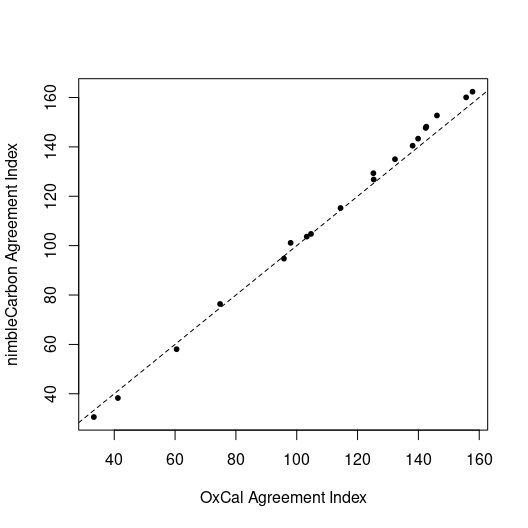1. This took approximately 20 minutes on a i7-7560U linux machine with 16G of RAM